#Factoring over the Complex NumbersNow you'll see mathematicians at work: making easy things harder to make them easier!

#### The Grandfather of all examples.

Consider the polynomial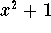. It cannot be factored over the real numbers, since its graph has no x-intercepts. (The graph is just the standard parabola shifted up by one unit!)

How can we tell that the polynomial is irreducible, when we perform square-completion or use the quadratic formula? Let's try square-completion: Not much to complete here, transferring the constant term is all we need to do to see what the trouble is: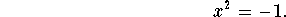We can't take square roots now, since the square of every real number is non-negative!

Here is where the mathematician steps in: She (or he) imagines that there are roots of -1 (not real numbers though) and calls them i and -i. So the defining property of this imagined number i is that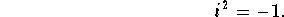Now the polynomialhas suddenly become reducible, we can write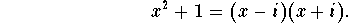#### Complex Numbers.

Let's get organized: A number of the form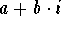, where a and b are real numbers, is called a complex number. Here are some examples: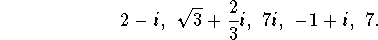The number a is called the real part of a+bi, the number b is called the imaginary part of a+bi.

Luckily, algebra with complex numbers works very predictably, here are some examples: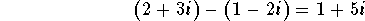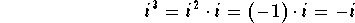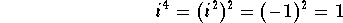In general, multiplication works with the FOIL method: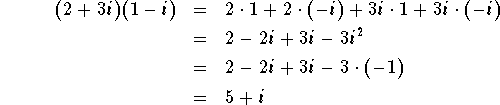Two complex numbers a+bi and a-bi are called a complex conjugate pair. The nice property of a complex conjugate pair is that their product is always a non-negative real number: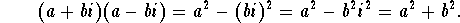Using this property we can see how to divide two complex numbers. Let's look at the example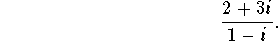The magic trick is to multiply numerator and denominator by the complex conjugate companion of the denominator, in our example we multiply by 1+i: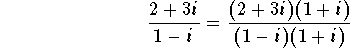Since (1+i)(1-i)=2 and (2+3i)(1+i)=-1+5i, we get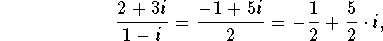and we are done!

#### Quadratic polynomials with complex roots.

Consider the polynomial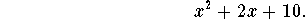Using the quadratic formula, the roots compute to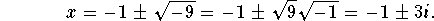It is not hard to see from the form of the quadratic formula, that if a quadratic polynomial has complex roots, they will always be a complex conjugate pair!

Here is another example. Consider the polynomialIts roots are given by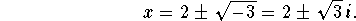#### The discriminant.

We can see from the graph of a polynomial, whether it has real roots or is irreducible over the real numbers. How can we tell algebraically, whether a quadratic polynomial has real or complex roots? The symbol i enters the picture, exactly when the term under the square root in the quadratic formula is negative. This term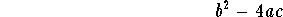is called the discriminant.

Consider the discriminant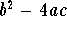of the quadratic polynomial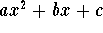.

• If the discriminant is positive, the polynomial has 2 distinct real roots.
• If the discriminant is negative, the polynomial has 2 complex roots, which form a complex conjugate pair.
• If the discriminant is zero, the polynomial has one real root of multiplicity 2.

#### The Fundamental Theorem of Algebra, Take Two.

We already know that every polynomial can be factored over the real numbers into a product of linear factors and irreducible quadratic polynomials. But now we have also observed that every quadratic polynomial can be factored into 2 linear factors, if we allow complex numbers. Consequently, the complex version of the The Fundamental Theorem of Algebra is as follows:

 Over the complex numbers, every polynomial (with real-valued coefficients) can be factored into a product of linear factors.

We can state this also in root language:

 Over the complex numbers, every polynomial of degree n (with real-valued coefficients) has n roots, counted according to their multiplicity.

The usage of complex numbers makes the statements easier and more "beautiful"!

#### Exercise 1.

Find all (real or complex) roots of the polynomial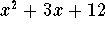.

#### Exercise 2.

Factor the polynomial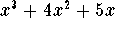completely (a) over the real numbers, (b) over the complex numbers.

#### Exercise 3.

For which values of c does the polynomial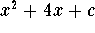have two complex conjugate roots?

#### Exercise 4.

For which values of a does the polynomial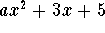have two distinct real roots?

#### Exercise 5.

Every quadratic polynomial has either 2 distinct real roots, one real root of multiplicity 2, or 2 complex roots. What cases can occur for a polynomial of degree 3? Give an example for each of these cases.

#### Exercise 6.

(a) Show that every polynomial of degree 3 has at least one x-intercept.

(b) Give an example of a polynomial of degree 4 without any x-intercepts.

#### Exercise 7.

Give an example of a polynomial of degree 5, whose only real roots are x=2 with multiplicity 2, and x=-1 with multiplicity 1.[Back] [Next]
[Algebra] [Trigonometry] [Complex Variables]
[Calculus] [Differential Equations] [Matrix Algebra]S.O.S MATHematics home page

Do you need more help? Please post your question on our S.O.S. Mathematics CyberBoard.Helmut Knaust